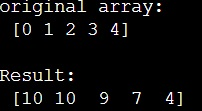# How to perform a reverse cumulative sum on a NumPy array?

Given a NumPy array, we have to perform a reverse cumulative sum on it.
Submitted by Pranit Sharma, on March 13, 2023

## NumPy Array - Performing a reverse cumulative sum

The cumulative sum for an array [1,2,3,4] can be defined as [1, 1+2, 1+2+3, 1+2+3+4] i.e., [1,3,6,10].

However, reverse cumulative is done in such a way that first all the elements are added in inverse order followed by the sum of all the elements in reverse except the first element followed by the sum of all the elements in reverse except 1st two elements until only the last element is left.

For example, for an array [1,2,3,4], the reverse cumulative sum would be [4+3+2+1, 4+3+2, 4+3, 4] i.e., [10,9,7,4].

In NumPy, we can simply find the reverse cumulative sum on an array using the indexing technique in reverse order (: :- 1) and apply cumsum on this.

Let us understand with the help of an example,

## Python code to perform a reverse cumulative sum on a NumPy array

```# Import numpy
import numpy as np

# Creating a numpy array
arr = np.array([0,1,2,3,4])

# Display original array
print("original array:\n",arr,"\n")

# Performing reverse cumulative sum
res = np.cumsum(arr[::-1])[::-1]

# Display result
print("Result:\n",res)
```

### OutputLanguages: » C » C++ » C++ STL » Java » Data Structure » C#.Net » Android » Kotlin » SQL
Web Technologies: » PHP » Python » JavaScript » CSS » Ajax » Node.js » Web programming/HTML
Solved programs: » C » C++ » DS » Java » C#
Aptitude que. & ans.: » C » C++ » Java » DBMS
Interview que. & ans.: » C » Embedded C » Java » SEO » HR
CS Subjects: » CS Basics » O.S. » Networks » DBMS » Embedded Systems » Cloud Computing
» Machine learning » CS Organizations » Linux » DOS
More: » Articles » Puzzles » News/Updates